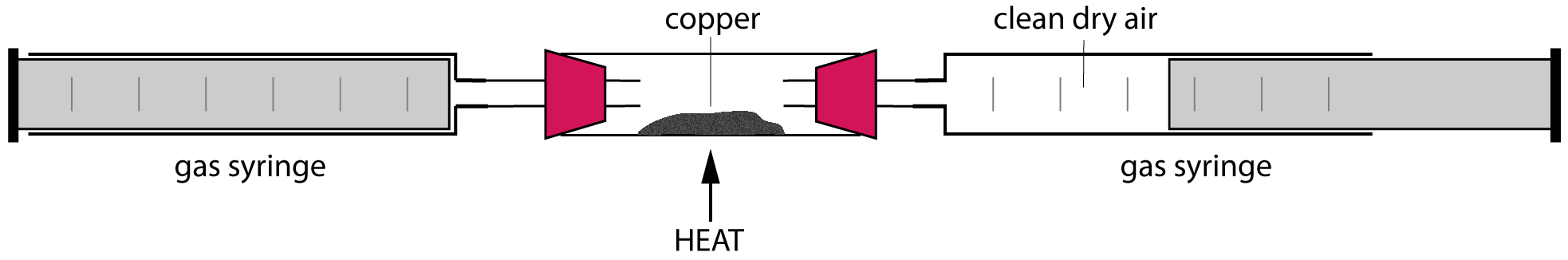Chemistry Edexcel iGCSE:
Gases + Pollutants

Page 1

1. The pie chart shows the approximate percentage of the three most abundant gases in dry air.

The gases represented by X, Y and Z are:X Y Z A oxygen carbon dioxide nitrogen B nitrogen Carbon dioxide oxygen C oxygen argon nitrogen D nitrogen argon oxygen
2. The test for oxygen gas is …
• A.   relights a glowing splint
• B.   ignites with a pop
• C.   turns limewater milky
• D.   a lit splint continues to burn

Q3-5:
A student set up the following apparatus to determine the percentage of oxygen in polluted air. He left the apparatus set up until the water level stopped rising and remained constant.

He obtained the following results:

• Initial volume of gas in the tube = 150 cm3
• Final volume of gas in the tube = 122 cm33. The percentage of oxygen, to the nearest whole number, in the polluted air is..
• A.   81%
• B.   28%
• C.   21%
• D.   19%
4. What would be the effect, if any, on the calculated percentage of oxygen in the air if the student left the experiment set up for longer?
5. What would be the effect, if any, on the calculated percentage of oxygen in the air if the student did not use excess iron wool?

6. Sulfur was burned in oxygen and the gas produced was added to water and tested with universal indicator (UI).

The correct balanced equation for this reaction and colour of universal indicator are:

 Balanced Equation Colour of UI solution A S + O2 → SO2 blue B S + O2 → SO2 red C 2S + O2 → 2SO blue D 2S + O2 → 2SO red

7. Observations made when magnesium burns in air include..

 Observations A Bright white flame White powder forms B Bright white flame Black powder forms C orange flame White powder forms D orange flame Black powder forms
Q8-9:
A sample of clean, dry air is passed over hot copper until all the oxygen in the air has reacted with the copper forming copper(II)oxide.8. What is the correct equation for the reaction occurring?
• A. 2Cu(s) +   O2(g)  2CuO(s)
• B.  Cu(s) +   O2(g)  CuO2(s)
• C. 4Cu(s) +   O2(g)  2Cu2O(s)
• D. 2Cu(s) +   O2(g)  Cu2O2(s)
9. If the volume of air decreased by 20 cm3 what was the starting volume of the air?
• A.   40 cm3
• B.   60 cm3
• C.   100 cm3
• D.   200 cm3